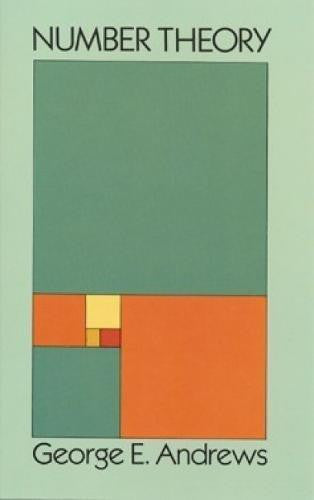\$ 14.95

Although mathematics majors are usually conversant with number theory by the time they have completed a course in abstract algebra, other undergraduates, especially those in education and the liberal arts, often need a more basic introduction to the topic.
In this book the author solves the problem of maintaining the interest of students at both levels by offering a combinatorial approach to elementary number theory. In studying number theory from such a perspective, mathematics majors are spared repetition and provided with new insights, while other students benefit from the consequent simplicity of the proofs for many theorems.
Among the topics covered in this accessible, carefully designed introduction are multiplicativity-divisibility, including the fundamental theorem of arithmetic, combinatorial and computational number theory, congruences, arithmetic functions, primitive roots and prime numbers. Later chapters offer lucid treatments of quadratic congruences, additivity (including partition theory) and geometric number theory.
Of particular importance in this text is the author's emphasis on the value of numerical examples in number theory and the role of computers in obtaining such examples. Exercises provide opportunities for constructing numerical tables with or without a computer. Students can then derive conjectures from such numerical tables, after which relevant theorems will seem natural and well-motivated..